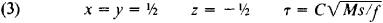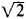# Dimensional Analysis

(redirected from Dimensionalysis)

## Dimensional analysis

A technique that involves the study of dimensions of physical quantities. Dimensional analysis is used primarily as a tool for obtaining information about physical systems too complicated for full mathematical solutions to be feasible. It enables one to predict the behavior of large systems from a study of small-scale models. It affords a convenient means of checking mathematical equations. Finally, dimensional formulas provide a useful cataloging system for physical quantities.

All the commonly used systems of units in physical science have the property that the number representing the magnitude of any quantity (other than purely numerical ratios) varies inversely with the size of the unit chosen. Thus, if the length of a given piece of land is 300 ft, its length in yards is 100. The ratio of the magnitude of 1 yd to the magnitude of 1 ft is the same as that of any length in feet to the same length in yards, that is, 3. The ratio of two different lengths measured in yards is the same as the ratio of the same two lengths measured in feet, inches, miles, or any other length units. This universal property of unit systems, often known as the absolute significance of relative magnitude, determines the structure of all dimensional formulas. See Units of measurement

In defining a system of units for a branch of science such as mechanics or electricity, certain quantities are chosen as fundamental and others as secondary, or derived. The choice of the fundamental units is always arbitrary and is usually made on the basis of convenience in maintaining standards. In mechanics the fundamental units most often chosen are mass, length, and time.

It can be proved that every secondary quantity which satisfies the condition of the absolute significance of relative magnitude is expressible as a product of powers of the primary quantities. Such an expression is known as the dimensional formula of the secondary quantity. There is no requirement that the exponents be integral.

The technique of dimensional analysis has several important applications. It is intuitively obvious that only terms whose dimensions are the same can be equated. The equation 10 kg = 10 m/s, for example, makes no sense. A necessary condition for the correctness of any equation is that the two sides have the same dimensions. This is often a help in the verification of complicated analytic expressions. Of course, an equation can be correct dimensionally and still be wrong by a purely numerical factor.

The application of dimensional analysis to the derivation of unknown relations depends upon the concept of completeness of equations. An expression which remains formally true no matter how the sizes of the fundamental units are changed is said to be complete. Assume a group of n physical quantities x1, x2, …, xn, for which there exists one and only one complete mathematical expression connecting them, namely, &phgr;(x1, x2, …, xn) = 0. Some of the quantities x1, x2, …, xn may be dimensional constants. Assume further that the dimensional formulas of the n quantities are expressed in terms of m fundamental quantities α, β, γ,…. Then it will always be found that this single relation &phgr; can be expressed in terms of some arbitrary function F of n - m independent dimensionless products π1, π2, …, πn-m, made up from among the n variables, as in the equation below.This is known as the π theorem. It was first rigorously proved by E. Buckingham. The main usefulness of the π theorem is in the deduction of the form of unknown relations. The procedure is particularly useful in hydraulics and aeronautical engineering, where detailed solutions are often extremely complicated.

A further application of dimensional analysis is in model design. Often the behavior of large complex systems can be deduced from studies of small-scale models at a great saving in cost. In the model each parameter is reduced in the same proportion relative to its value in the original system.

Dimensional formulas also provide a convenient shorthand notation for representing the definitions of secondary quantities and are helpful in changing units from one system to another.

## Dimensional Analysis

a method of establishing the relationship between the physical quantities that are of importance in a given phenomenon. It is based on an examination of the dimensions of the quantities.

A fundamental requirement of dimensional analysis is that the equation expressing the desired relationship must remain valid under any change in the units of the quantities occurring in it. An equivalent requirement is that the dimensions on the left and right sides of the equation must be the same. The dimensional formula of a physical quantity has the form

(1) [N] = LlMmTt

Here, [N] is a symbol for the dimension of a secondary, or derived, quantity; it is usually written with brackets. L, M, T, … are symbols for the quantities taken as the fundamental quantities and denote length, mass, and time, respectively. The exponents l, m, t, … are positive or negative integral or fractional real numbers. The set of the exponents is called the dimensions of the secondary quantity [N]. Thus, the dimensional formula for acceleration a is written as [a] = LT-2, and that for force as [F] = LMT-2.

The concept of dimensions can be extended to fundamental quantities. It is assumed that the exponent of the fundamental quantity in this case is unity and that the quantity is independent of other quantities. The dimensional formula for a fundamental quantity thus coincides with the quantity’s symbol. If the unit of a derived quantity does not change when one of the fundamental units is changed, the dimension of the derived quantity in that fundamental quantity is zero. Thus, in the dimensional formula for acceleration, mass has the exponent zero. When the exponents of all the fundamental quantities in a dimensional formula are zero, the quantity associated with the formula is said to be dimensionless. The choice of the number of physical quantities taken as fundamental is theoretically arbitrary, as is the choice of the quantities themselves. Practical considerations, however, result in some limitation of the freedom to choose the fundamental quantities and the units of the fundamental quantities.

In the cgs system of units, the fundamental quantities are length, mass, and time. In this system, a dimensional formula is expressed by the product of the three symbols L, M, and T with the appropriate exponents. The International System of Units has seven fundamental quantities.

Suppose that for a given phenomenon an unknown quantity can be shown to be related to certain other quantities, although the form of this relation is unknown. It is then possible to construct a dimensional equation on whose left side is the symbol for the unknown quantity together with its exponent and on whose right side is the product of the symbols of the quantities of which the unknown quantity is a function; the exponents on the right side are unknown. The relation between the physical quantities in this case can be determined by finding the values of the quantities’ exponents. For example, if we wish to determine the time τ that a body of mass M moving forward in a straight line under the action of the constant force f requires to travel a distance s, we can form the dimensional equation

(2) T = Lxmy (LMT-2)z

where x, y, and z are unknowns. Since the exponents on the left and right sides of equation (2) must be equal for each quantity, we obtain the system of equations x + z = 0, y + z = 0, and — 2z = 1. Consequently,The dimensionless coefficient C, which according to the laws of mechanics is equal to, cannot be determined within the framework of dimensional analysis. This fact exemplifies the distinctive feature of a dimensional analysis. The relation this method establishes between the unknown quantity and the quantities determining the phenomenon under investigation may be in error by a constant coefficient or a coefficient that is a function of a dimensionless parameter, for example, an angle. Additional data are required to obtain exact quantitative relations. Dimensional analysis is not, therefore, an all-purpose method. It has been fruitfully applied in those fields of physics, such as hydraulics and aerodynamics, where an exact solution of a problem is often extraordinarily difficult to obtain, particularly because of the large number of parameters that may determine a given physical phenomenon.

A major role in the solution of complex problems by means of dimensional analysis is played by the π theorem, according to which every relation between a particular number of dimensional quantities characterizing a given physical phenomenon can be represented in the form of a relation between a lesser number of dimensionless combinations of these quantities. This theorem links dimensional analysis with the theory of physical similarity, which is based on the assertion that two physical phenomena are physically similar if all the corresponding dimensionless characteristic quantities for the phenomena are identical (seeSIMILARITY THEORY).

### REFERENCES

Bridzhmen, P. V. Analiz razmernostei. Leningrad-Moscow, 1934.
Sedov, L. I. Metody podobiia i razmernosti v mekhanike, 6th ed. Moscow, 1967.
Kogan, B. Iu. Razmernost’ fizicheskoi velichiny. Moscow, 1968.
Sena, L. A. Edinitsy fizicheskikh velichin i ikh razmernosti. Moscow, 1969.

L. A. SENA

## dimensional analysis

[də′men·chən·əl ə′nal·ə·səs]
(physics)
A technique that involves the study of dimensions of physical quantities, used primarily as a tool for obtaining information about physical systems too complicated for full mathematical solutions to be feasible.
Site: Follow: Share:
Open / Close